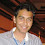## Saturday, November 10, 2012

### Fun with FEniCS, Part 3

That's the same heat equation, except now on a circular domain rather than a square. However, more interesting visualization can be done if we add an extra time derivative,

utt - ∆u = 0,

which is the wave equation. For a circular domain, it models vibrations of a drumhead. Here it is, with an initial condition that looks like the horseshoe condition but smoothed out by the previous heat equation (I saved the data and used it as initial data in the hyperbolic equation):

Generally, it was a lot more computationally intensive to do this instead of the heat equation, as I had to use a smaller timestep. Also, the general time scale on which the dynamics becomes interesting is longer. I wrote to file only once per 10 timesteps (otherwise I would have had 12000 frames, which would have given a video 3 minutes and 20 seconds long!). As for computational speed, using FEniCS was definitely a boon; if I had generated this in MATLAB, it would have easily taken a whole day. The circular equations is a great way to talk about Bessel functions, which will definitely make an appearance here someday. But suffice to say, their use was not necessary here.

1.This comment has been removed by the author.

2.This comment has been removed by the author.

3.No problem. This is blog is mostly about testing out some well-established algorithms in current research in numerical analysis, learning how to use various finite element software, and recording my observations (and indeed, demystifying a lot of the math and showing that even simple concepts lead to pretty good visualizations). Yes, I indeed used Paraview to show it, and the code is an extended version of the FEniCS tutorial's example (they do in fact have an example of doing dynamics). Quite a bit has changed in FEniCS since I wrote this post a few years ago, so I'm going to have to dig it out in my hard drive and refresh and/or test it a bit. I'll keep in contact via email. Glad you liked these simulations!

4.This comment has been removed by the author.

5.This comment has been removed by the author.